• Slides: 27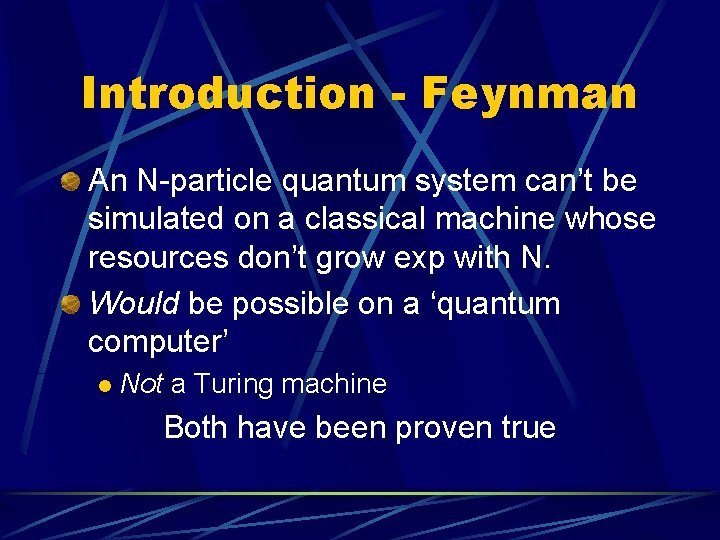Introduction - Feynman An N-particle quantum system can’t be simulated on a classical machine whose resources don’t grow exp with N. Would be possible on a ‘quantum computer’ l Not a Turing machine Both have been proven trueIntroduction, cont’d Quantum parallelism – quantum superposition of distinct states l Doesn’t immediately lead to speedup Shor showed how info could be extracted usefully l Polynomial factoring algo.Intro to ……. . On a classical computer, unsorted database search takes O(n) time In 1997, Grover showed a quantum algo that takes O( sqrt N )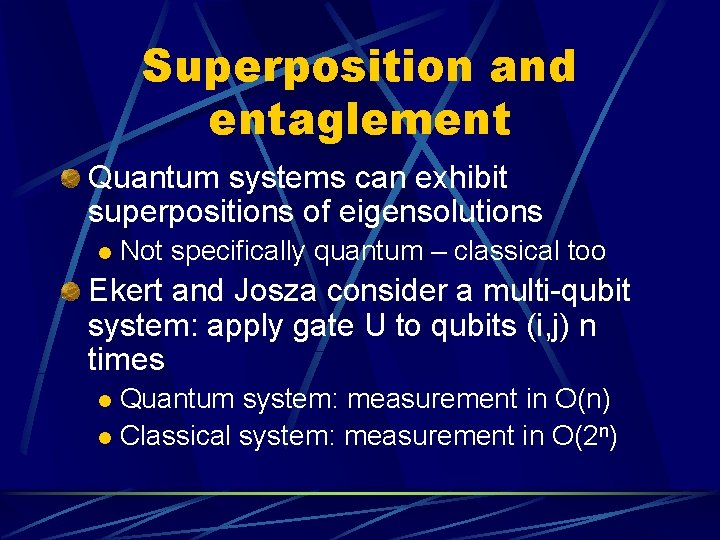Superposition and entaglement Quantum systems can exhibit superpositions of eigensolutions l Not specifically quantum – classical too Ekert and Josza consider a multi-qubit system: apply gate U to qubits (i, j) n times Quantum system: measurement in O(n) l Classical system: measurement in O(2 n) l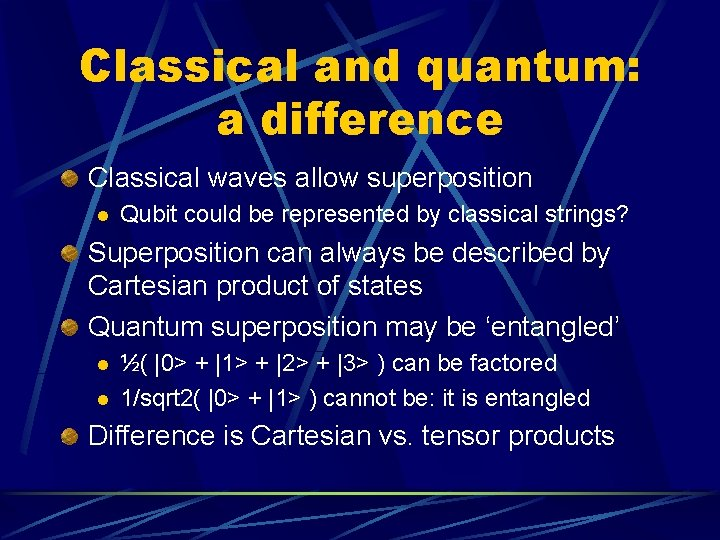Classical and quantum: a difference Classical waves allow superposition l Qubit could be represented by classical strings? Superposition can always be described by Cartesian product of states Quantum superposition may be ‘entangled’ l l ½( |0> + |1> + |2> + |3> ) can be factored 1/sqrt 2( |0> + |1> ) cannot be: it is entangled Difference is Cartesian vs. tensor productsEntanglement, cont’d Schroedinger says quantum entanglement is defining characteristic Entanglement depends on basis l ½( |0> + |1> - |2> + |3> ) is entangled wrt C 2 x C 2, but not wrt C 4 State of n qubits is 2 n-dim, isomorphic to 1 particle with 2 n levels. l Not useful for complexity consideration, as the 1 particle requires energy resources in O( 2 n )Back to Grover Search through a phone book for name, only knowing telephone number Takes O(n) time classically l O( sqrt n ) time by Grover’s algo l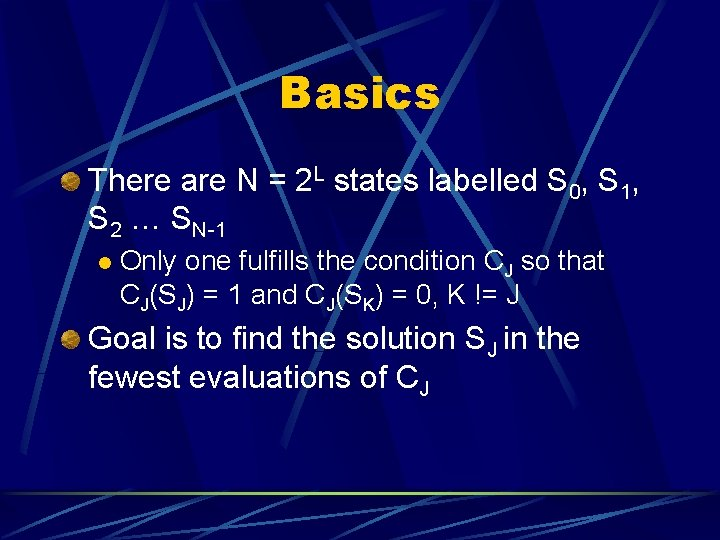Basics There are N = 2 L states labelled S 0, S 1, S 2 … SN-1 l Only one fulfills the condition CJ so that CJ(SJ) = 1 and CJ(SK) = 0, K != J Goal is to find the solution SJ in the fewest evaluations of CJ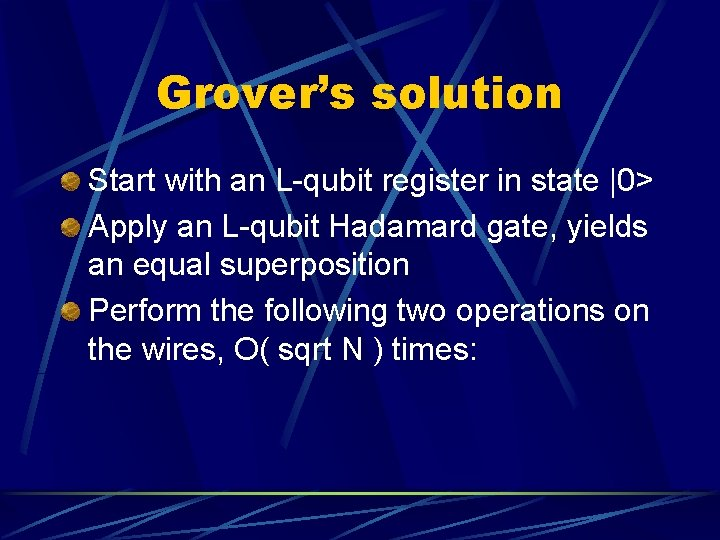Grover’s solution Start with an L-qubit register in state |0> Apply an L-qubit Hadamard gate, yields an equal superposition Perform the following two operations on the wires, O( sqrt N ) times:Grover’s operations 1) Apply oracle UJ defined by: UJ |J> = -|J> l UJ |K> = |K>, K != J l 2) Apply diffusion operator D: D = H U 0 H l U 0|0> = -|0> l U 0|K> = -|K>, K != 0 lResult After O( sqrt N ) iterations, the outcome is the state |J> with high probability Grover explains D to be an ‘inversion about the average’ of the coefficients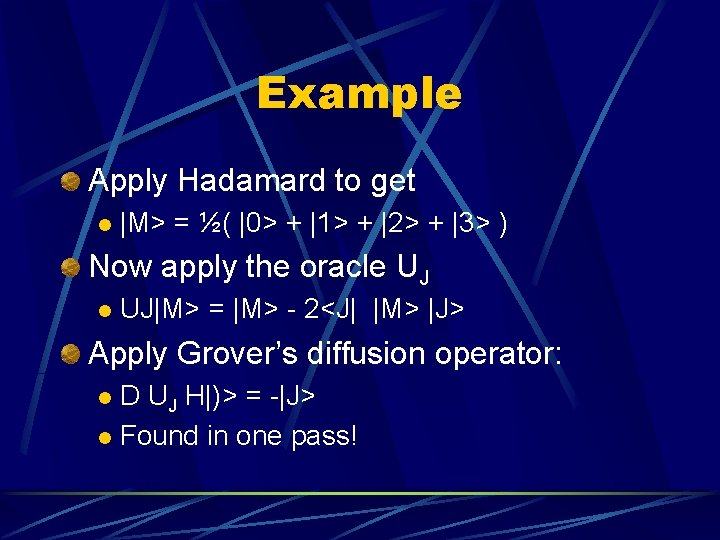Example Apply Hadamard to get l |M> = ½( |0> + |1> + |2> + |3> ) Now apply the oracle UJ l UJ|M> = |M> - 2<J| |M> |J> Apply Grover’s diffusion operator: D UJ H|)> = -|J> l Found in one pass! lClassical implementation We map each integer 0… 2 L-1 into another integer in the same range: Define L qubits to be a ‘control’ register |J> and another L to be the ‘target’ register |K> l Let |J> x |K> |J> x |K x f(J)> l Starting with |K> = 0, we get |f(J)> lClassical, cont’d Consider an f(M) that maps an integer M to an integer F = f(M) (bijective) Want to force init state into |M> x |F(M)> so that we can measure f-1(F) = M Define W = Vf Hc l Let Uf be an oracle that flips the sign of the state iff it is |F> l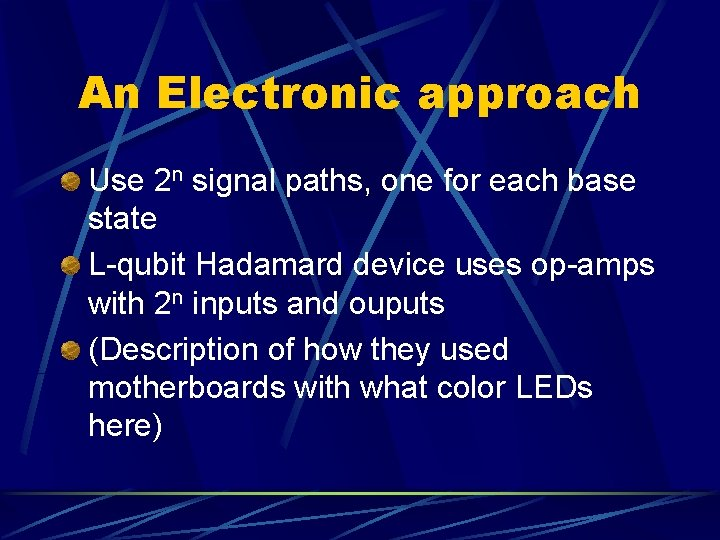An Electronic approach Use 2 n signal paths, one for each base state L-qubit Hadamard device uses op-amps with 2 n inputs and ouputs (Description of how they used motherboards with what color LEDs here)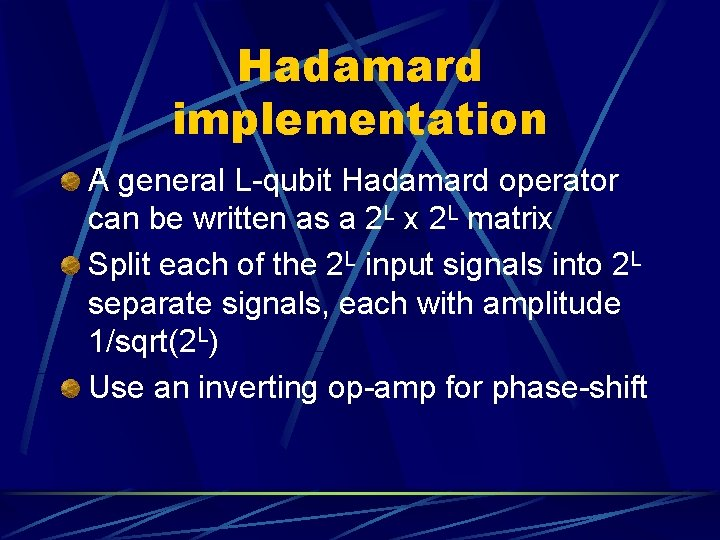Hadamard implementation A general L-qubit Hadamard operator can be written as a 2 L x 2 L matrix Split each of the 2 L input signals into 2 L separate signals, each with amplitude 1/sqrt(2 L) Use an inverting op-amp for phase-shift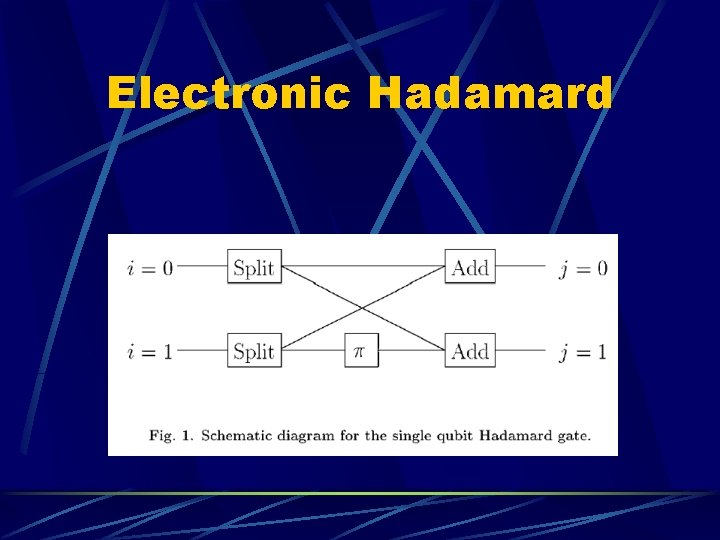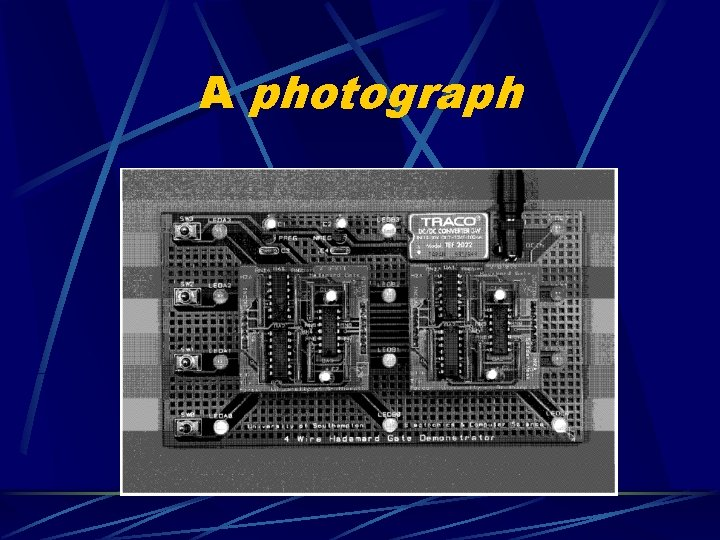A photograph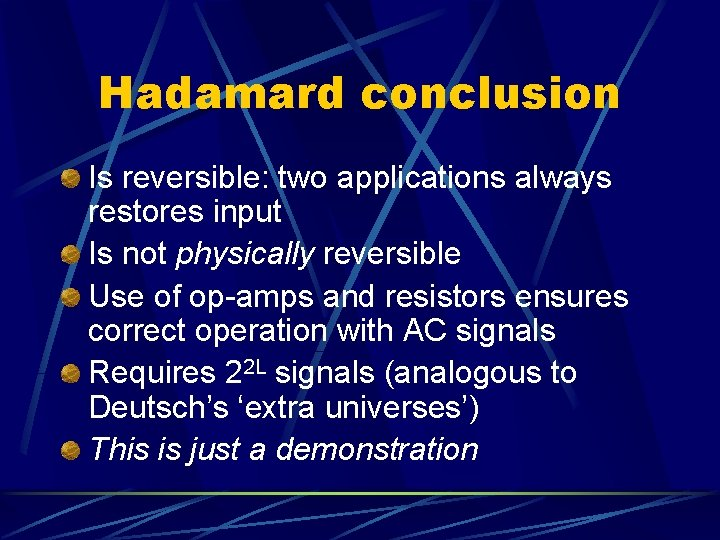Hadamard conclusion Is reversible: two applications always restores input Is not physically reversible Use of op-amps and resistors ensures correct operation with AC signals Requires 22 L signals (analogous to Deutsch’s ‘extra universes’) This is just a demonstrationGrover schematic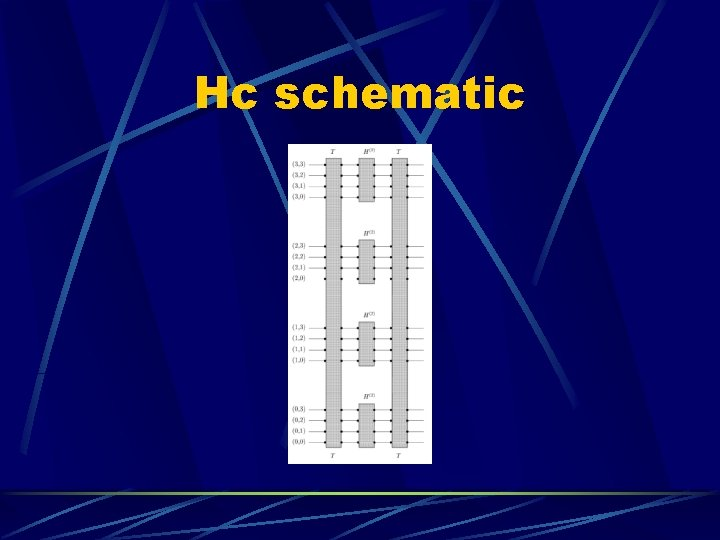Hc schematicT matrix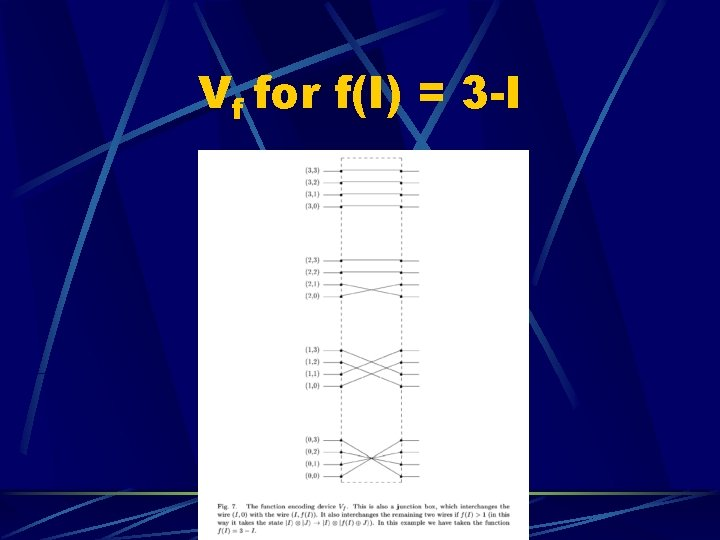Vf for f(I) = 3 -I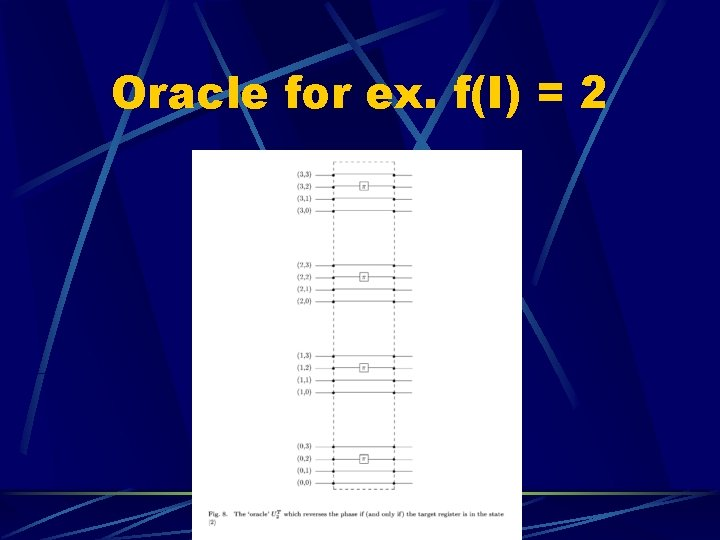Oracle for ex. f(I) = 2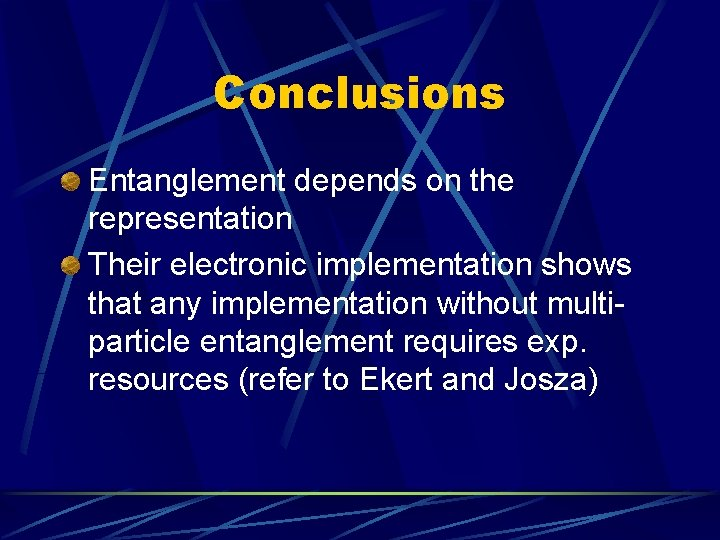Conclusions Entanglement depends on the representation Their electronic implementation shows that any implementation without multiparticle entanglement requires exp. resources (refer to Ekert and Josza)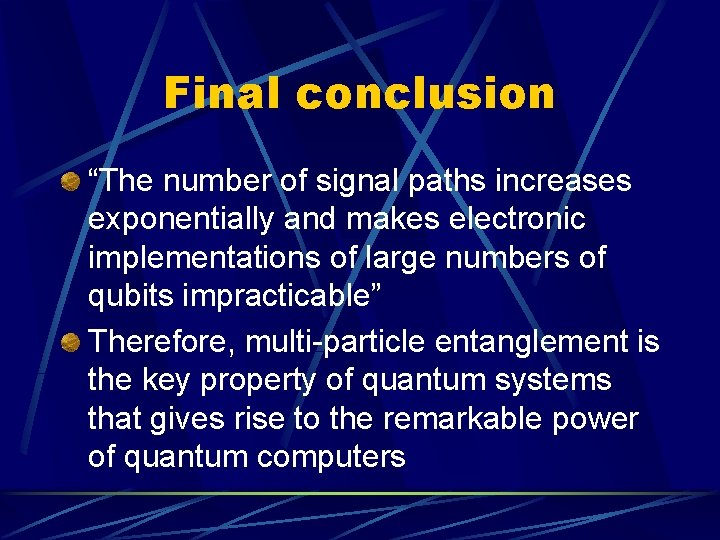Final conclusion “The number of signal paths increases exponentially and makes electronic implementations of large numbers of qubits impracticable” Therefore, multi-particle entanglement is the key property of quantum systems that gives rise to the remarkable power of quantum computers